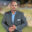Related Tags

javascript

# What are the spread and rest operators in javascript?Kedar Kodgire

In Javascript, we use the spread operator to split up array elements or object properties.

Hence, the name spread, i.e., “spread the elements of an array/objects in a new array/objects”.

### Example 1

An example of a spread operator with arrays is as follows:

const numbers = [1, 2, 3, 4]

const numbers_with_spread = [...numbers, 5, 6]

const numbers_without_spread = [numbers, 5, 6]

console.log(numbers_without_spread)

### Explanation

• Line 1: We will initiate the numbers array.
• Line 3: We create a new array numbers_with_spread using the spread operator.
• Line 5: We create another array numbers_without_spread without using the spread operator.
• Line 7–9: We will print the new arrays to the console.

### Example 2

An example of spread operator using objects is as follows:

const human = {
name : "Kedar"
}

...human,
age : '24'
}

human,
age : '24'
}

console.log(human_age_without_spread)

### Explanation

• Line 1-3: We will initiate the human object.
• Line 5-8: We create a new object human_age_with_spread using the spread operator.
• Line 10-13: We start another object human_age_without_spread, without using the spread operator.
• Line 16 –17: We will print the new arrays to the console.

### Overview: rest

It's a collection of remaining elements. We use it for merging a list of functional arguments into an array, i.e., we can use this when we don't know how many arguments are passed to our method.

Hence, the name rest, i.e., “the rest of the elements”.

### Example

const more_than_three = (...args) => {
console.log(args)  /* lets see what's in args */
return console.log(args.filter((x) => x > 3))
}

more_than_three(1,2,3,4,5,6,7,8)

### Explanation

• Line 1: We define a function more_than_three() using the rest operator in the arguments.
• Line 2: We will print the received arguments.
• Line 3: We will return the numbers that are greater than 3.
• Line 6: We will make a function call.

Note: The syntax for spread and rest is the same (...), but use-cases differ.

RELATED TAGS

javascript

CONTRIBUTORKedar Kodgire
RELATED COURSES

View all Courses

Keep Exploring

Learn in-demand tech skills in half the time